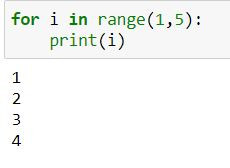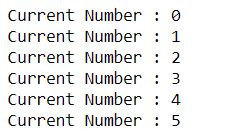Search
•Ekta Aggarwal

# Loops in Python

There are two loop in python which we shall be studying in detail in this tutorial:

### While loops

While loops are entry-controlled loops which means they test for a condition first. If the condition is true then only the loop is executed otherwise the loop will terminate.

Syntax:

```while(condition to be tested):
<Do something>
else:
<Do something>```

Let us create a temporary variable:

`temp = 5`

Following while loop checks if the value of temp is less than or equal to 10 or not. If temp <= 10 then the loop is executed.

```while(temp <=10):
print(temp);
temp+=1;```

temp +=1 means temp = temp+1 i.e. increment the value of temp by 1.

For temp = 5, while will get executed, it will print 5 and increment temp by 1 i.e. temp will now be equal to 6.

Now for temp = 6, loop will be executed and temp will be incremented to 7.

When temp = 11 the condition will get false and the loop will be terminated.### while - else

We can also define an else clause with while loop which will be executed at the time of exiting the loop

```temp = 5;
while(temp <=10):
print(temp);
temp+=1;
else: print('Exiting the loop at temp = '+ str(temp))```

At temp = 11 while loop will be terminated and will move to the else part.You can also ad if else conditions inside a while loop.

In the following example when temp = 7 we are printing 7 is my lucky number, otherwise for all other values it will just print the number.

```temp = 5;
while(temp <=10):
if temp == 7: print('7 is my lucky number')
else: print(temp)
temp+=1;
else: print('Exiting the loop at temp = '+ str(temp))```### For loop

Unlike for loop we do not need to specify an increment statement (like temp+=1 in case of while). For loop automatically increments the iterator_variable by 1.

Syntax:

```for iterator_variable in iterable:
<Do something>```

### Iterating over lists

Let us create a list:

`my_list = ['Washington D.C.','London','Tokyo','Moscow']`

In the following for loop, our iterator variable is i and we are iterating over each element of our list:

```for i in my_list:
print(i)```

Firstly i takes the value "Washington D.C." and prints it, then i takes the value "London" and it gets printed until it reaches the end of the list.

### Iterating over range

range(x,y) is like a list starting from x till y-1 (not y is excluded) Eg. range(1,5) is actually a list [1,2,3,4]

```for i in range(1,5):
print(i)```range(x) is a list starting from 0 and containing elements till x-1 i.e. range(5) is a list [0,1,2,3,4]

```for i in range (5):
print(i)```range(x,y,by) is a list starting from x till y-1 where numbers are incremented with 'by' . Eg.

range(1,10,2) = [1,3,5,7,9]

```for i in range(1,10,2):
print(i)```### Nested for loop:

A for loop inside a for loop is called nested for loop.

In the following code firstly i takes the value 1 and iterates over all the elements of my_list (i.e. j).

Then i = 2 and all the elements of my_list get printed and so on.

```for i in range(1,5):
for j in my_list:
print(i,j)```For different iterables for loops get slightly modified. To learn more you can refer to this detailed tutorial: For loop for different iterables.

### Break statement

break statement can be used with both for loop and while loops. Whenever a loop encounters a break statement then all the loops (if-else conditions, for loops, while loops, nested loops) would be terminated.

```for mynum in range(50):
if (mynum > 5):
break
print('Current Number :', mynum)```

In the above code, as soon as mynum takes the value 6 break statement gets trigerred and our loop's execution get terminated. Thus we have values printed only till mynum = 5.### Continue statement

continue statement can be used with both for loop and while loops. Whenever a loop encounters a continue statement then Python moves the control to the beginning of the loop - it won't break the loop but will not run further commands and will increment the value of the iterator.

```for mynum in range(10):
print('Current Number :', mynum) #first print statement
if (mynum > 5):
continue
print('Next Number would be :', mynum+1) #second print statement```

In the above code, when mynum is <=5 then current and next number get printed, but as soon as mynum takes the value 6 continue statement gets trigerred - which means the next number (second print statement) won't be executed. and mynum would be incremented to 7 and the loop would continue.See All